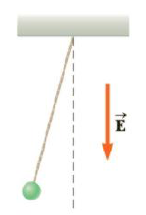Chapter 15, Problem 62AP

Chapter
Section
Textbook Problem

A 1.00-g cork ball having a positive charge of 2.00 mC is suspended vertically on a 0.500-m-long light siring in the presence of a uniform downward-directed electric field of magnitude E = 1.00 × 105 N/C as in Figure P15.62. If the ball is displaced slightly from the vertical, it oscillates like a simple pendulum. (a) Determine the period of the ball’s oscillation, (b) Should gravity be included in the calculation for part (a)? Explain.Figure P15.62

(a)

To determine
The period of ball’s acceleration.

Explanation

Given info: Mass of the cork ball (m) is 1.00 g. Charge on the ball (q) is 2.00 mC. Length of the string (l) is 0.500 m. The magnitude of electric field (E) is 1.00×105N/C .

Formula to calculate the time period is,

T=2πlg

• g is the acceleration.

Formula to calculate the acceleration is,

g=qE+magm=qEm+ag

• ag is the acceleration due to gravity.

Therefore,

T=2πlqEm+ag

Substitute 1.00 g for m, 2.00 mC for q, 0.500 m for l and 9.8ms-2 for ag.

T=2π0.500m(1

(b)

To determine
The effect of gravity on time period.

Still sussing out bartleby?

Check out a sample textbook solution.

See a sample solution

The Solution to Your Study Problems

Bartleby provides explanations to thousands of textbook problems written by our experts, many with advanced degrees!

Get Started

Find more solutions based on key concepts